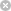RTXI  2.1fir_dsgn.h
Go to the documentation of this file.
1 /*
2  * Functions for class FirFilterDesign
3  */
4
5 #ifndef _FIR_DSGN_H_
6 #define _FIR_DSGN_H_
7
8 #include "typedefs.h"
9 #include "gen_win.h"
10
11 typedef enum {
17 } FIR_SYM_T;
18
20 {
21 public:
22  // default constructor
23
25
26  // constructor that allocates array of length num_taps to hold coefficients
27
28  FirFilterDesign(int num_taps);
29
30  // constructor that allocates array of length num_taps and initializes impulse
31  // response
32
33  FirFilterDesign(int num_taps, double* imp_resp_coeff);
34
35  // constructor for filter with symmetric impulse response given half the
36  // coefficients
37
38  FirFilterDesign(int num_taps, FIR_SYM_T symmetry, double* imp_resp_coeff);
39
40  // allocate coefficient array *Imp_Resp_Coeff after default constructor
41
42  void Initialize(int num_taps);
43
44  // method to scale coefficients
45
46  void ScaleCoefficients(double scale_factor);
47
48  // scale coefficients so that magnitude response has unity gain at passband
49  // peak
50
51  void NormalizeFilter(void);
52
53  // set impulse response to values in input array
54
55  void CopyCoefficients(double* coeff);
56
57  double* GetCoefficients(void);
58
59  // get number of filter taps
60
61  int GetNumTaps(void);
62
63  // apply discrete-time window to filter coefficients
64
65  void ApplyWindow(GenericWindow* window);
66
67  // extract a subset of coefficient values for defining
68  // a polyphase filter
69
70  void ExtractPolyphaseSet(double* coeff, int decim_rate, int rho);
71
72 protected:
73  int Num_Taps;
74
75  double* Imp_Resp_Coeff;
76  double* Original_Coeff;
77  long* Quant_Coeff;
79 };
80
81 #endif
FIR_SYM_T
Definition: fir_dsgn.h:11
void ScaleCoefficients(double scale_factor)
Definition: fir_dsgn.cpp:85
double * Imp_Resp_Coeff
Definition: fir_dsgn.h:75
long * Quant_Coeff
Definition: fir_dsgn.h:77
void ApplyWindow(GenericWindow *window)
Definition: fir_dsgn.cpp:138
void NormalizeFilter(void)
Definition: fir_dsgn.cpp:99
FIR_SYM_T Coeff_Symmetry
Definition: fir_dsgn.h:78
double * GetCoefficients(void)
Definition: fir_dsgn.cpp:130
int GetNumTaps(void)
Definition: fir_dsgn.cpp:124
void ExtractPolyphaseSet(double *coeff, int decim_rate, int rho)
Definition: fir_dsgn.cpp:147
void CopyCoefficients(double *coeff)
Definition: fir_dsgn.cpp:115
void Initialize(int num_taps)
Definition: fir_dsgn.cpp:75
double * Original_Coeff
Definition: fir_dsgn.h:76